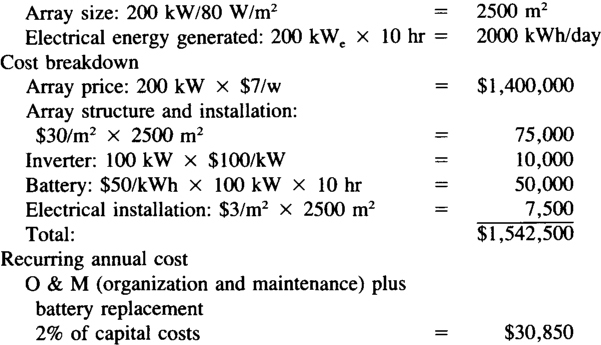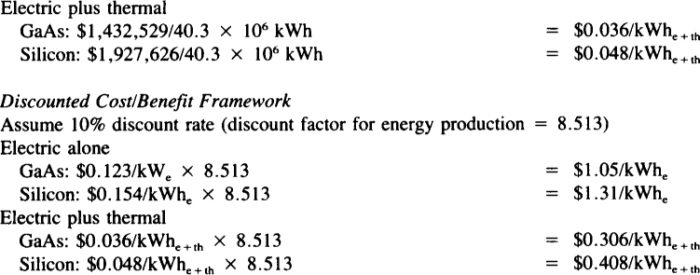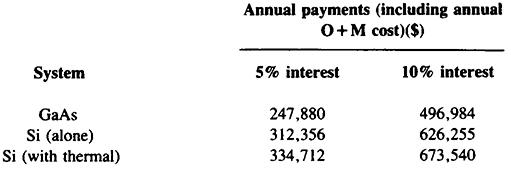# Solar Energy System Cost

Want create site? Find Free WordPress Themes and plugins.

A general method of calculation will start from the load energy requirements; determine the local insolation level and the worst month insolation and from this determine the sizing of the storage capacity. From this follows the array power and area, the battery capacity, and eventually the voltage regulator size respectively, and the inverter/converter size.

The insolation in kilowatt-hour per square meters per day is known for most areas of the Earth. The average value of kilowatt-hour per square meters over a day is roughly one half the maximum value (around 1 kW/m2) times the hours of insolation. This value has to be averaged again to obtain the yearly average of insolation. Typical mean values from winter to summer in northern regions of the U.S. vary from 3 to 6 kWh/m2 day. Detailed values exist for all of the U.S. They vary from 2.3 in northern regions to 6.5 in desert regions like Arizona.

In a detailed analysis, the required array power is calculated by dividing the energy load value, L (kilowatt hours per day), by the array-sizing factor, S (kilowatt hours per square meters day), and a number of dimensionless factors accounting for the converter, battery, degradation, etc. For example, L = 2.8 kWh/day and S is 3.7 kWh/m2day the ratio L/S would give the required number of square meters, here, 0.756 m2. Due to loss factors, this value is generally multiplied by 1.The value of s = 3.7 kWh/m2day means that lm2 delivers 3.7 kWh/day to the solar cells. The actual output of the solar cells depends on their efficiency.

A normal panel with 10% conversion efficiency will thus deliver only 0.37 kWh/ day. A division of the L/S value (times 1.3) by the panel efficiency and the factors taking care of temperature degradation results in a value

$A=\frac{{L}/{S}\;}{\eta \left[ 1+{{P}_{tc}}\left( {{T}_{op}}-{{28}^{o}}C \right) \right]}$

Where Ptc is the temperature degrading factor, and Top is the operating temperature. With an efficiency of 10% of the solar cells and a temperature degrading factor of Ptc = 0.005 and a top temperature of 55°C, the actual area for a value of L/S = 0.756 m2 (or 756 Wp for a normal insolation of 1 kWp/m2 which has to be set at 1063 W in most cases) will result in an area of: A = 12 to 13 m2 instead of 10 m2 for the average daily energy requirement of 2.8 kWh.

In the following, we will compare a silicon flat-plate solar array and an III-V-compound (GaAsAl/GaAs) solar cell system with concentrator and cogeneration.

## Basic Systems Assumptions

1. 100 kWe array for day use
2. 100 kWe array of batteries for night use## Silicon System Costs## GaAs-System CostsCapital Investment Requirements## Capital Recovery Loan

Assume: 20-year payback of principal and interest## Annual Energy Costs## Annual Energy Utilized

Electric: 584,000 kWhe

Thermal: 1,430,800 kWhth

Total: 2,014,800 kWhe+th

Did you find apk for android? You can find new Free Android Games and apps.